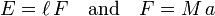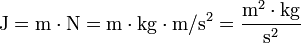# Joule (unit)

The joule (symbol J) is the SI unit of energy—a measure of the capacity to do work or generate heat. One joule equals the work done (or energy expended) by a force of one newton (N) acting over a distance of one meter (m). One newton equals a force that produces an acceleration of one meter per second (s) per second on a one kilogram (kg) mass. Therefore, one joule equals one newton•meter.

Expressed in equations:$E = \ell\, F \quad\hbox{and}\quad F = M\, a$

The mechanical quantities involved have the SI units:$E\; [\mathrm{J}], \quad \ell\; [\mathrm{m}], \quad F\; [\mathrm{N}], \quad M\; [\mathrm{kg}], \quad a\; [\mathrm{m}/\mathrm{s}^2].$

It follows that J (joule) is expressed in SI basic units as:$\mathrm{J} = \mathrm{m}\cdot\mathrm{N} = \mathrm{m}\cdot \mathrm{kg}\cdot \mathrm{m}/ \mathrm{s}^{2} = \frac{\mathrm{m}^2\cdot \mathrm{kg}}{\mathrm{s^2}}$.

'Joule' is variously pronounced 'jool' (rhymes with 'jewel') or 'joul' (rhymes with 'jowl').

One may also use electric units to define the joule. One joule measures the energy released by a electric charge of one coulomb dropping one volt in absolute value of electrical potential. The amount of energy delivered by a one watt source of power in one second is one Joule. The joule is also used to measure thermal energy. One calorie of heat is the equivalent of 4.186 J.

The joule is named for James Prescott Joule (1818 - 1889), who studied the relation between mechanical and heat energy discovered earlier by count Rumford.

One joule represents a relatively small amount of energy; it takes roughly 100,000 J (105 J) to heat a cup of water from room temperature to its boiling point under standard conditions. Often, kilojoules (kJ) are used, kJ = 103 J.

## Other units of energy convertible to joules

Whereas the joule is the standard SI unit of energy, many other units of energy, all convertible to joules, are used in particular circumstances. Those include: erg; foot pound; watt second; watt hour; kilowatt hour; thermochemical calorie; International Table calorie; kilocalorie; thermochemical Btu; therm; quad; cubic meter natural gas; ton of oil equivalent; ton of coal equivalent; ton of TNT; electron volt; hartree; inverse meter; inverse second; kelvin; kilogram. One kilogram (kg) of mass is equivalent to exactly 89,875,517,873,681,764 joules (according to E=mc2 with m = 1 kg and speed of light c = 299,792,458 m/s).

## Practical examples

• The amount of energy one utilizes in lifting a cup of water from the table to one's mouth equals approximately 1 Joule.
• One calorie (1 1/1000 of a kilocarie of food consumed or metabolic energy expended) equals 4.186 J.
• A baseball pitched by a fast Major League pitcher has a kinetic energy of approximately 230 J.
• One "dietary Calorie" (as used in the United States to designate a kilocalorie) is 4186 J (4.186 kJ).
• One gram of gasoline releases about 50 kJ on combustion in air.
• A small car (about 1200 kg) travelling at 65 miles per hour (105 km/h) has a kinetic energy of 1 megajoule (1 MJ).
• One "kilowatt-hour" is 3.6 MJ.
• One ton of TNT releases 4.184 GJ on exploding.

## References cited

1. 1.0 1.1 Elert G. (1998-2008) Energy: The Physics Hypertextbook™. A Work in Progress. Fair Use Encouraged.
2. 2.0 2.1 Foland AD. (2007). Energy. Chelsea House Publications. ISBN 978-0791089309.Some content on this page may previously have appeared on Citizendium.